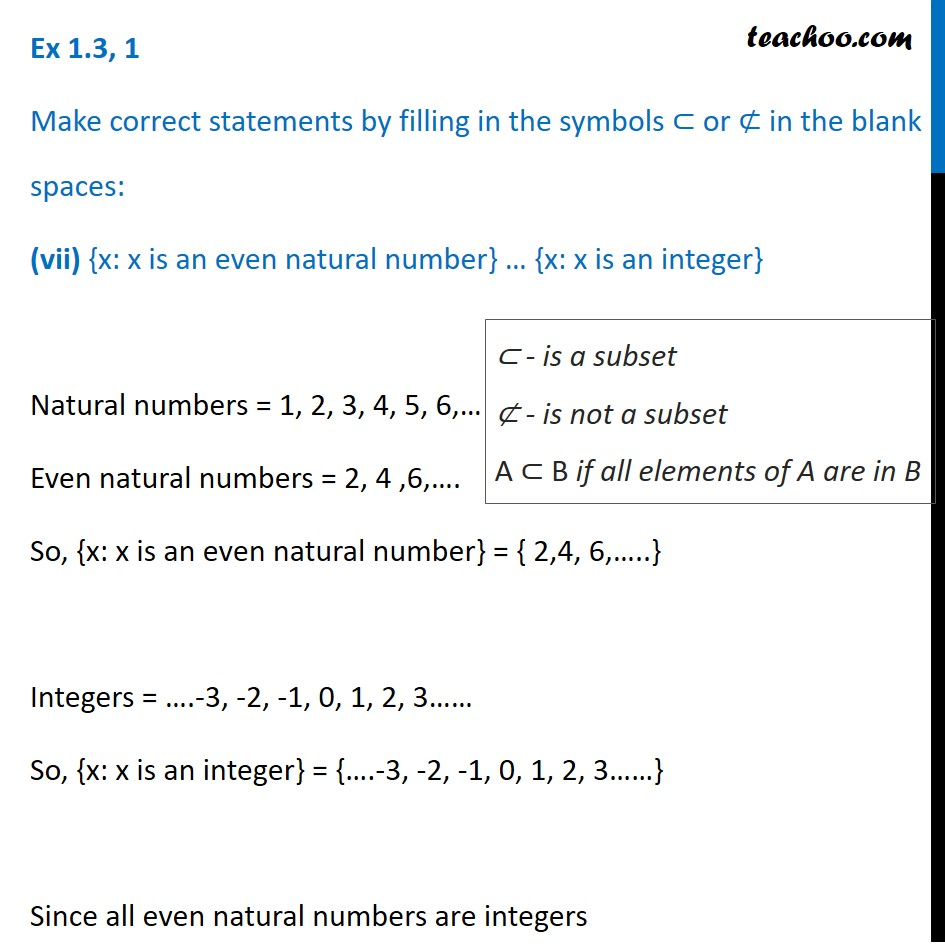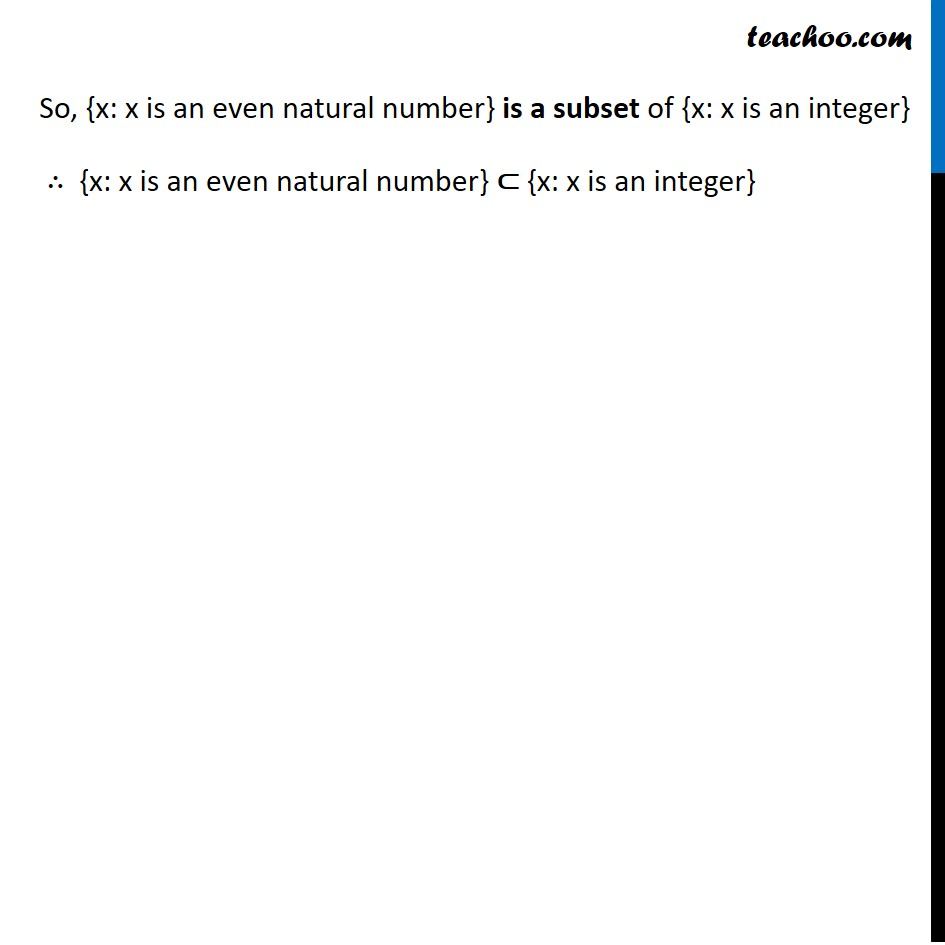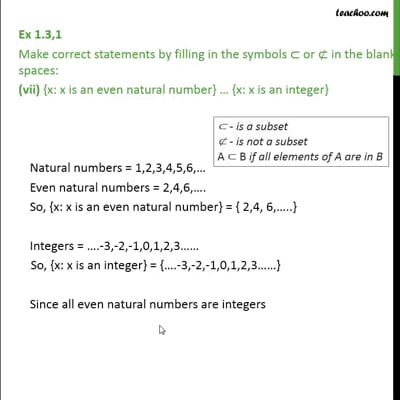Ex 1.3

Chapter 1 Class 11 Sets
Serial order wiseThis video is only available for Teachoo black users

Introducing your new favourite teacher - Teachoo Black, at only ₹83 per month

### Transcript

Ex 1.3, 1 Make correct statements by filling in the symbols ⊂ or ⊄ in the blank spaces: (vii) {x: x is an even natural number} … {x: x is an integer} Natural numbers = 1, 2, 3, 4, 5, 6,… Even natural numbers = 2, 4 ,6,…. So, {x: x is an even natural number} = { 2,4, 6,…..} Integers = ….-3, -2, -1, 0, 1, 2, 3…… So, {x: x is an integer} = {….-3, -2, -1, 0, 1, 2, 3……} Since all even natural numbers are integers ⊂ - is a subset ⊄ - is not a subset A ⊂ B if all elements of A are in B So, {x: x is an even natural number} is a subset of {x: x is an integer} ∴ {x: x is an even natural number} ⊂ {x: x is an integer}